Q-Chem 5.0 User’s Manual

# A.7 GDIIS

Direct inversion in the iterative subspace (DIIS) was originally developed by Pulay for accelerating SCF convergence . Subsequently, Csaszar and Pulay used a similar scheme for geometry optimization, which they termed GDIIS . The method is somewhat different from the usual quasi-Newton type approach and is included in Optimize as an alternative to the EF algorithm. Tests indicate that its performance is similar to EF, at least for small systems; however there is rarely an advantage in using GDIIS in preference to EF.

In GDIIS, geometries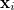generated in previous optimization cycles are linearly combined to find the “best” geometry on the current cycle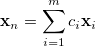(A.33)

where the problem is to find the best values for the coefficients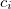.

If we express each geometry,, by its deviation from the sought-after final geometry,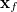, i.e.,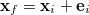, where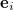is an error vector, then it is obvious that if the conditions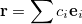(A.34)

and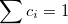(A.35)

are satisfied, then the relation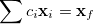(A.36)

also holds.

The true error vectorsare, of course, unknown. However, in the case of a nearly quadratic energy function they can be approximated by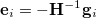(A.37)

where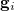is the gradient vector corresponding to the geometryand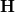is an approximation to the Hessian matrix. Minimization of the norm of the residuum vector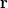, Eq. (A.34), together with the constraint equation, Eq. (A.35), leads to a system of (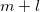) linear equations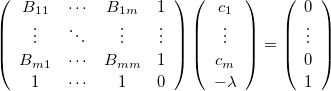(A.38)

where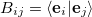is the scalar product of the error vectorsand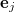, and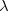is a Lagrange multiplier.

The coefficientsdetermined from Eq. (A.38) are used to calculate an intermediate interpolated geometry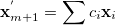(A.39)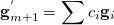(A.40)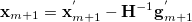(A.41)